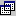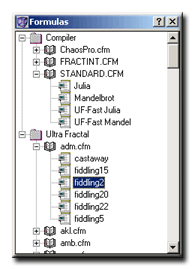### Assigning Formulas

 If you want to assign a formula to a fractal, you normally need to click onto some kind of button. After having pressed this button a window will appear (see below):This formula browser window displays all formulas which ChaosPro has found. The formula which currently is assigned to the fractal is initially selected. Expand and collapse the folders as needed until you have found the formula you would like to use. Then double click onto the formula name. The window will close and the formula will be used by the fractal. All parameter values for that formula will be set to the default values for that formula. Note: This window is a “focus window”, i.e. as soon as this window does not have the focus any more it will close automatically. If you hold down the SHIFT key on your keyboard then the parameter values are preserved. That means, if the old formula had a parameter called “perturb” and the new formula has a parameter with the same name, then the value of that parameter will be preserved. Normally (i.e. without pressing the shift key) all parameters are initialized with the default values for that formula.

Another way of assigning a formula is by using the Compiler window: That window also has a tree view control displaying all formulas. It works just the same: Double clicking onto a formula name assigns the formula.

If a formula cannot be found (which can happen if you load a parameter set), then the fractal will display a “not found: ” error text where normally the formula name will be displayed.